2019-06-20 12:04:09 weixin_31866177 阅读数 893
• ###### Kubernetes 实战系列

Kubernetes（KS8） 实战系列教程，该课程将对Kubernetes的Node、Pod、Replication Controller、Service等基本核心概念进行讲解，这些组件共同构成了Kubernetes的系统框架和计算模型。通过对它们进行灵活的组合，用户就可以快速、方便地对容器集群进行配置、创建和管理。

9522 人正在学习 去看看 CSDN讲师

## KS检验-风控角度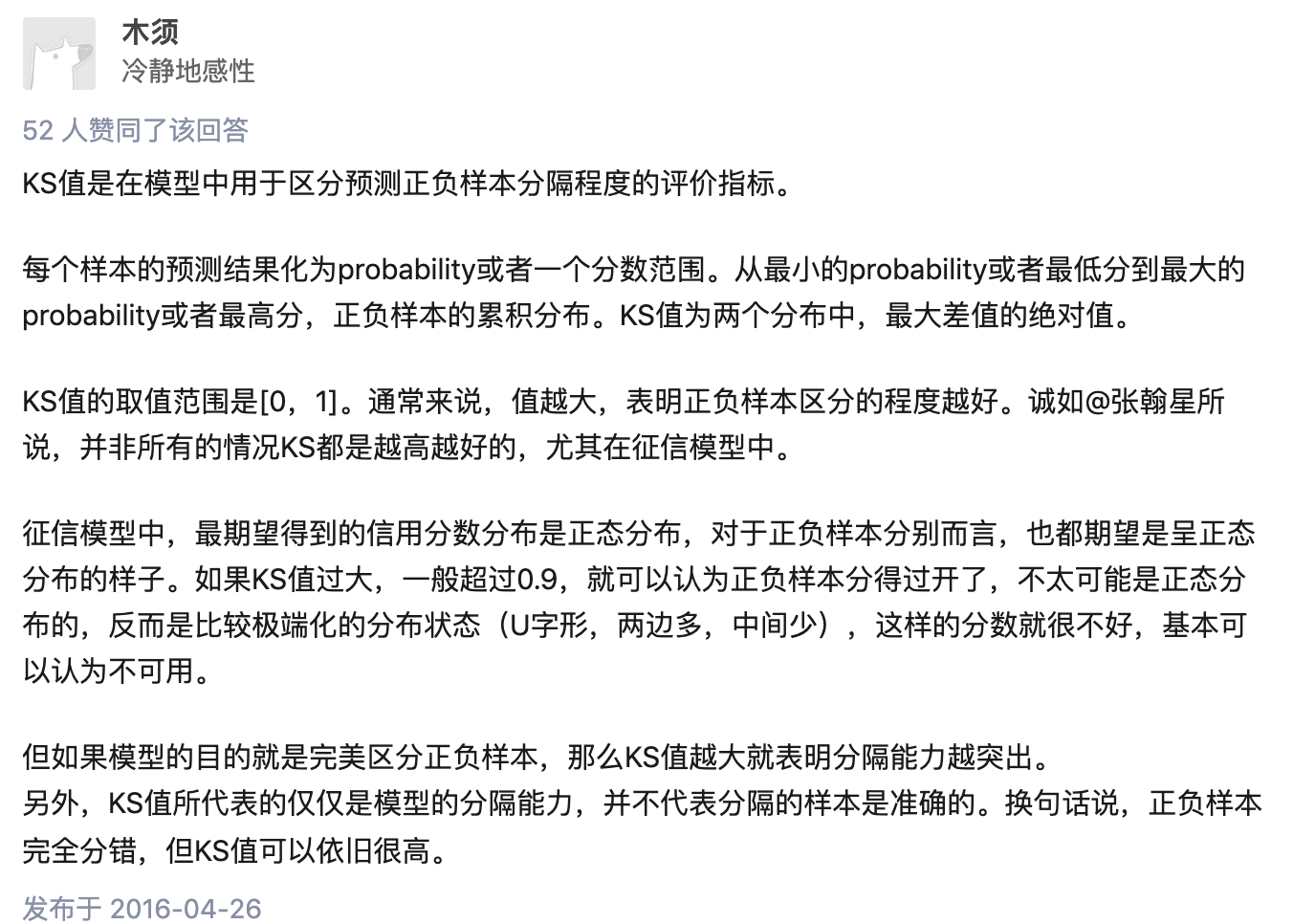def compute_ks(data1,data2):
df1 = DataFrame()
df1['pred'] = data2
df1['label'] = data1
# 按照样本为正样本的概率值升序排序，也即坏样本的概率从高到低排序
sorted_list = df1.sort_values(['pred'], ascending=[True])
# print(sorted_list)
"""
pred     label
17  0.055966    0.0
8   0.056266    0.0
14  0.063441    0.0
15  0.066217    0.0
0   0.070942    0.0
4   0.074102    0.0
3   0.087055    0.0
2   0.090387    0.0
18  0.092003    1.0
6   0.098286    0.0
16  0.105280    0.0
10  0.107256    0.0
12  0.123415    0.0
5   0.137829    0.0
7   0.139731    0.0
1   0.159905    0.0
13  0.180385    0.0
9   0.203199    0.0
11  0.217903    0.0
"""
total_good = sorted_list['label'].sum() # label为1的样本有多少个，真实为1的样本
# print(sorted_list['label'])
# print(total_good)
total_bad = sorted_list.shape - total_good # label为0的样本有多少个，真实为0的样本

max_ks = 0.0
good_count = 0.0
for index, row in sorted_list.iterrows(): #按照标签和每行拆开
# print(index)
# print('-'*5)
# print(row)
"""
index: 17
row:
pred     0.055966
label    0.000000
"""
if row['label'] == 0:
else:
good_count += 1
max_ks = max(max_ks, val)
return max_ks

def cal_auc(labels, preds):
"""
先排序，然后统计有多少正负样本对满足：正样本预测值>负样本预测值, 再除以总的正负样本对个数
复杂度 O(NlogN), N为样本数
"""
n_pos = sum(labels)
n_neg = len(labels) - n_pos
total_pair = n_pos * n_neg

labels_preds = zip(labels, preds)
labels_preds = sorted(labels_preds, key=lambda x: x)
accumulated_neg = 0
satisfied_pair = 0
for i in range(len(labels_preds)):
if labels_preds[i] == 1:
satisfied_pair += accumulated_neg
else:
accumulated_neg += 1

return satisfied_pair / float(total_pair)

def approximate_auc(labels, preds, n_bins=100):
"""
近似方法，将预测值分桶(n_bins)，对正负样本分别构建直方图，再统计满足条件的正负样本对
复杂度 O(N)
这种方法有什么缺点？怎么分桶？

"""
n_pos = sum(labels)
n_neg = len(labels) - n_pos
total_pair = n_pos * n_neg

pos_histogram = [0 for _ in range(n_bins)]
neg_histogram = [0 for _ in range(n_bins)]
bin_width = 1.0 / n_bins
for i in range(len(labels)):
nth_bin = int(preds[i] / bin_width)
if labels[i] == 1:
pos_histogram[nth_bin] += 1
else:
neg_histogram[nth_bin] += 1

accumulated_neg = 0
satisfied_pair = 0
for i in range(n_bins):
satisfied_pair += (pos_histogram[i] * accumulated_neg + pos_histogram[i] * neg_histogram[i] * 0.5)
accumulated_neg += neg_histogram[i]

return satisfied_pair / float(total_pair)

# official
def calc_ks(y_true, y_prob, n_bins=10):
percentile = np.linspace(0, 100, n_bins + 1).tolist()
bins = [np.percentile(y_prob, i) for i in percentile]
bins = bins - 0.01
bins[-1] = bins[-1] + 0.01
binids = np.digitize(y_prob, bins) - 1
y_1 = sum(y_true == 1)
y_0 = sum(y_true == 0)
bin_true = np.bincount(binids, weights=y_true, minlength=len(bins))
bin_total = np.bincount(binids, minlength=len(bins))
bin_false = bin_total - bin_true
true_pdf = bin_true / y_1
false_pdf = bin_false / y_0
true_cdf = np.cumsum(true_pdf)
false_cdf = np.cumsum(false_pdf)
ks_list = np.abs(true_cdf - false_cdf).tolist()
ks = max(ks_list)
return ks

# 在网上看其他人实现的方案，但是感觉是错的，和我们的问题可能有出入，
# 等之后再研究吧
from scipy.stats import ks_2samp
get_ks = lambda y_pred,y_true: ks_2samp(y_pred[y_true==1], y_pred[y_true!=1]).statistic
get_ks(x,y)
# mine
ks = ks_2samp(y, y_pred)
print("ks:",ks.statistic)

• FPRate的意义是所有真实类别为0的样本中，预测类别为1的比例。（好人为1）

f(good) 预测为负,真实为正除以所有真实正样本数。真正例率：

• TPRate的意义是所有真实类别为1的样本中，预测类别为1的比例。（坏人为0）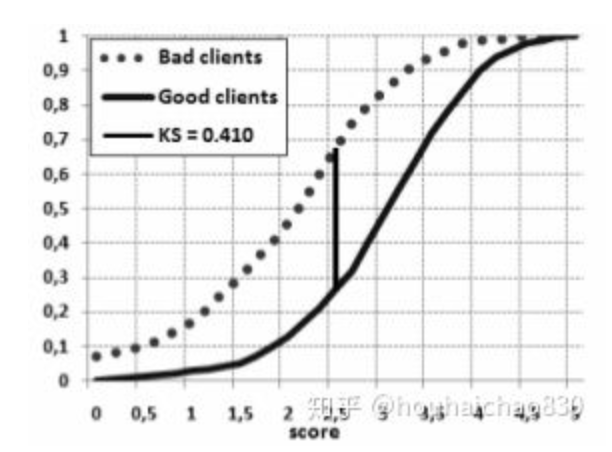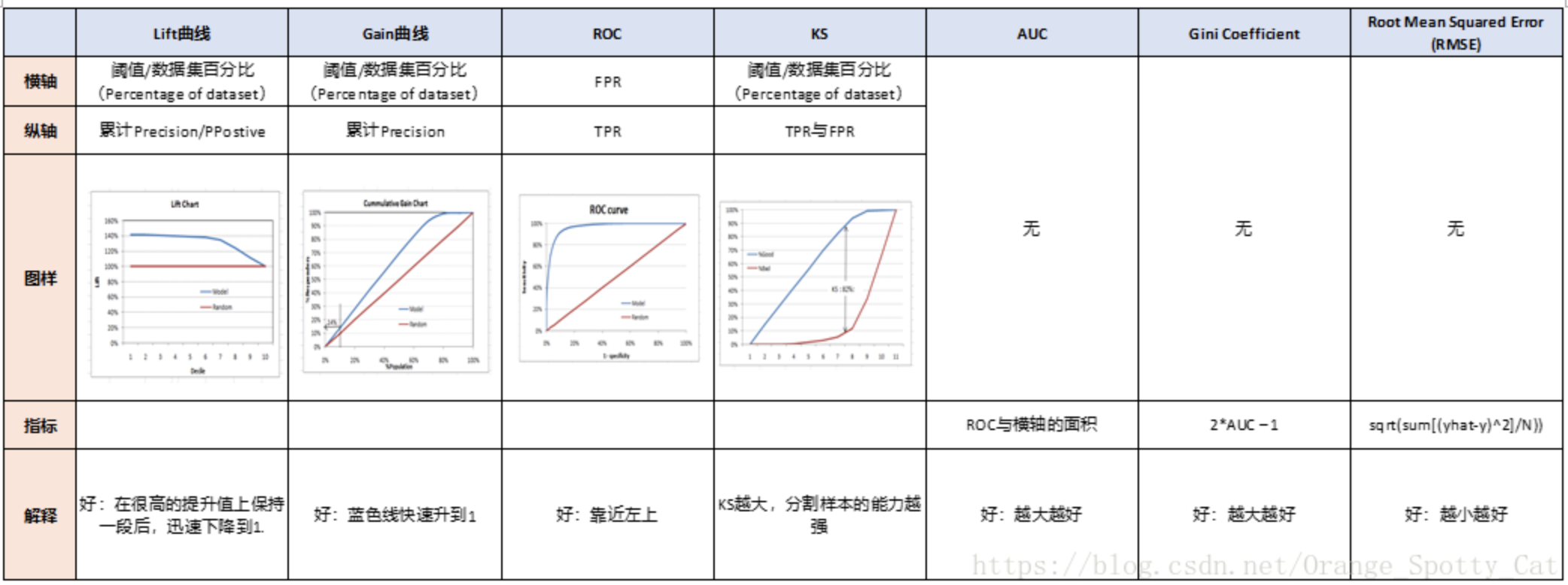def ks_2samp(data1, data2):
"""
Computes the Kolmogorov-Smirnov statistic on 2 samples.
This is a two-sided test for the null hypothesis that 2 independent samples
are drawn from the same continuous distribution.

data: 而为数组或dataframe，包括模型得分和真实的标签
score_col:一维数组或series，代表模型得分（一般为预测正类的概率）
class_col:一维数组或series，代表真实的标签（（0，1）or（-1，1））

ks：ks值
cdf_df:好坏人累积概率分布以及其差值gap

Parameters
----------
data1, data2 : sequence of 1-D ndarrays
two arrays of sample observations assumed to be drawn from a continuous
distribution, sample sizes can be different
Returns
-------
statistic : float
KS statistic
pvalue : float
two-tailed p-value
Notes
-----
This tests whether 2 samples are drawn from the same distribution. Note
that, like in the case of the one-sample K-S test, the distribution is
assumed to be continuous.
This is the two-sided test, one-sided tests are not implemented.
The test uses the two-sided asymptotic Kolmogorov-Smirnov distribution.
If the K-S statistic is small or the p-value is high, then we cannot
reject the hypothesis that the distributions of the two samples
are the same.
Examples
--------
>>> from scipy import stats
>>> np.random.seed(12345678)  #fix random seed to get the same result
>>> n1 = 200  # size of first sample
>>> n2 = 300  # size of second sample
For a different distribution, we can reject the null hypothesis since the
pvalue is below 1%:
>>> rvs1 = stats.norm.rvs(size=n1, loc=0., scale=1)
>>> rvs2 = stats.norm.rvs(size=n2, loc=0.5, scale=1.5)
>>> stats.ks_2samp(rvs1, rvs2)
(0.20833333333333337, 4.6674975515806989e-005)
For a slightly different distribution, we cannot reject the null hypothesis
at a 10% or lower alpha since the p-value at 0.144 is higher than 10%
>>> rvs3 = stats.norm.rvs(size=n2, loc=0.01, scale=1.0)
>>> stats.ks_2samp(rvs1, rvs3)
(0.10333333333333333, 0.14498781825751686)
For an identical distribution, we cannot reject the null hypothesis since
the p-value is high, 41%:
>>> rvs4 = stats.norm.rvs(size=n2, loc=0.0, scale=1.0)
>>> stats.ks_2samp(rvs1, rvs4)
(0.07999999999999996, 0.41126949729859719)
"""
data1 = np.sort(data1)
data2 = np.sort(data2)
n1 = data1.shape
n2 = data2.shape
data_all = np.concatenate([data1, data2])
cdf1 = np.searchsorted(data1, data_all, side='right') / (1.0*n1)
cdf2 = np.searchsorted(data2, data_all, side='right') / (1.0*n2)
d = np.max(np.absolute(cdf1 - cdf2))
# Note: d absolute not signed distance
en = np.sqrt(n1 * n2 / float(n1 + n2))
try:
prob = distributions.kstwobign.sf((en + 0.12 + 0.11 / en) * d)
except:
prob = 1.0

return Ks_2sampResult(d, prob)

houhaichao830

4.4.2分类模型评判指标（四） - ROC，AUC，GINI，KS，Lift，Gain，MSE总结

auc和ks是强相关的指标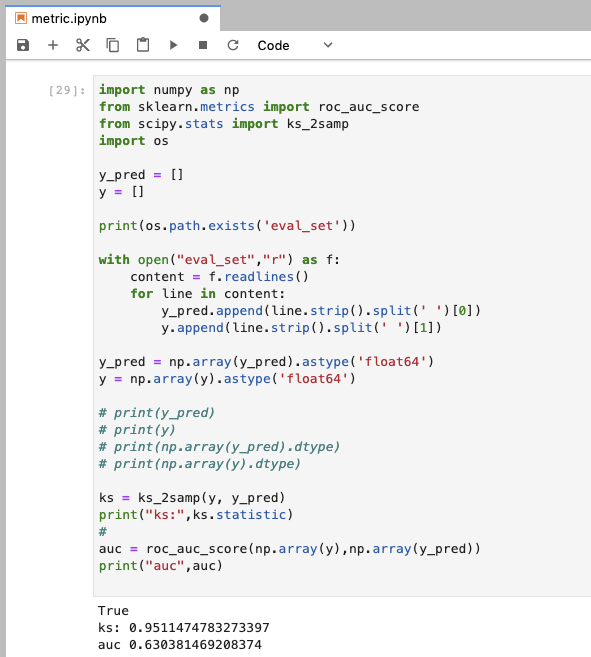astype转成一样的浮点数才可以进行减法操作！！！要dtype查看，之前这里报错辽~?

ks值 机器学习 相关内容

2018-12-06 16:22:35 zwqjoy 阅读数 8457
• ###### Kubernetes 实战系列

Kubernetes（KS8） 实战系列教程，该课程将对Kubernetes的Node、Pod、Replication Controller、Service等基本核心概念进行讲解，这些组件共同构成了Kubernetes的系统框架和计算模型。通过对它们进行灵活的组合，用户就可以快速、方便地对容器集群进行配置、创建和管理。

9522 人正在学习 去看看 CSDN讲师

# 背景介绍

Confusion Matrix -> Lift，Gain，ROC。

ROC -> AUC，KS -> GINI。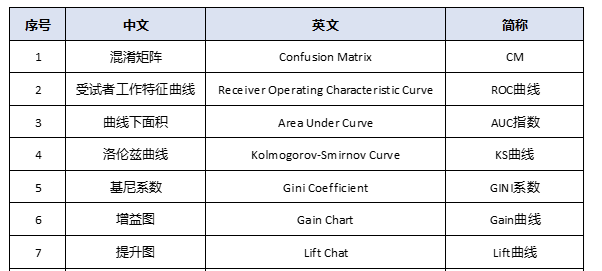1. 帮主是ROC曲线。

2. 副帮主是KS曲线，AUC面积

3. AUC养了一个小弟，叫GINI系数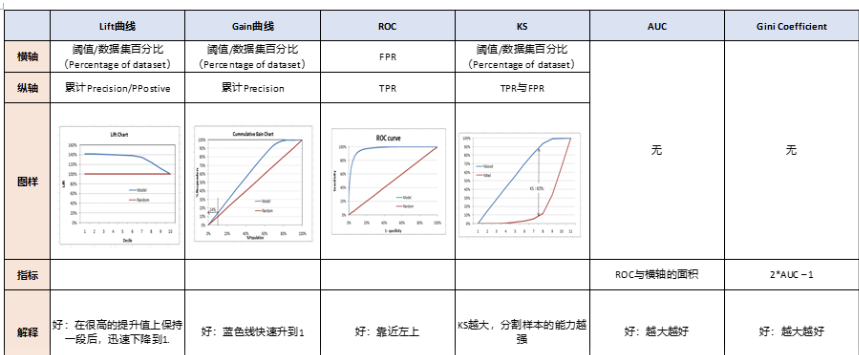# 二 KS曲线

KS曲线其实数据来源和本质和ROC曲线是一致的，只是ROC曲线是把真正率和假正率当作横纵轴，而K-S曲线是把真正率和假正率都当作是纵轴，横轴则由选定的阈值来充当。

KS(Kolmogorov-Smirnov)：KS用于模型风险区分能力进行评估，指标衡量的是好坏样本累计分部之间的差值。好坏样本累计差异越大，KS指标越大，那么模型的风险区分能力越强。

KS的计算步骤如下：
1. 计算每个评分区间的好坏账户数。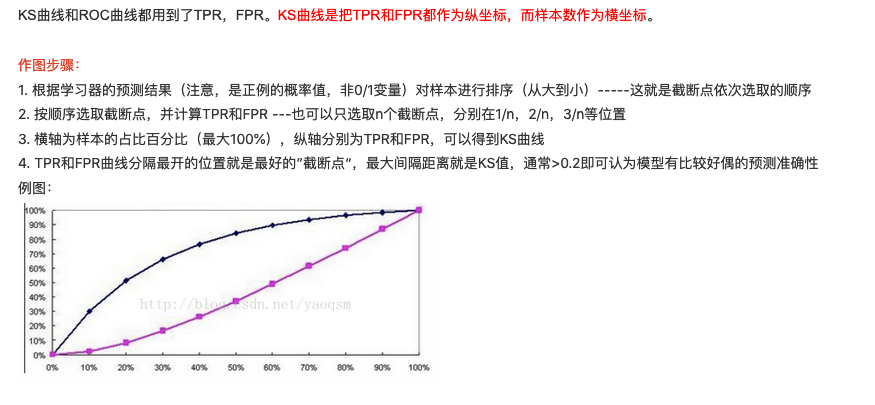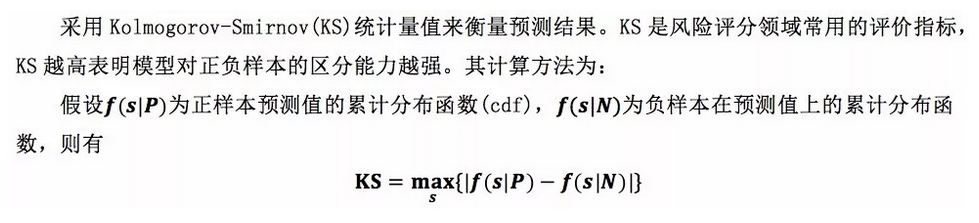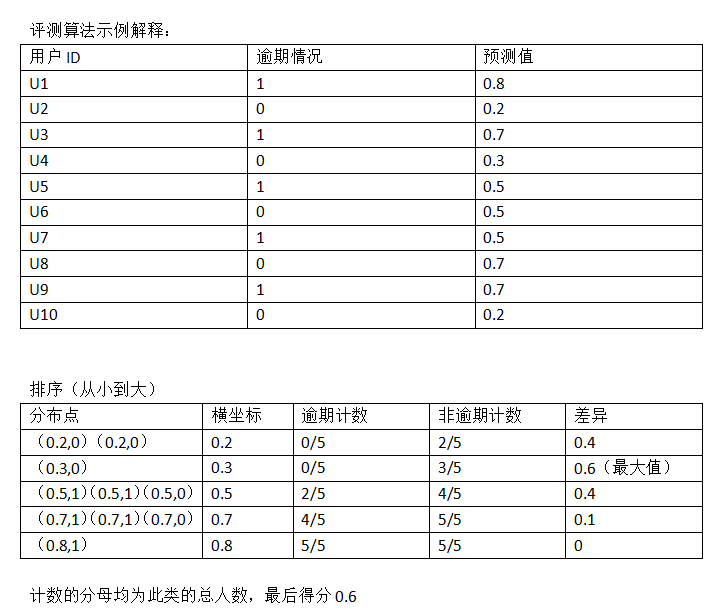https://blog.csdn.net/sinat_30316741/article/details/80018932

ROC值一般在0.5-1.0之间。值越大表示模型判断准确性越高，即越接近1越好。ROC=0.5表示模型的预测能力与随机结果没有差别。
KS值表示了模型将+和-区分开来的能力。值越大，模型的预测准确性越好。一般，KS>0.2即可认为模型有比较好的预测准确性。
KS值一般是很难达到0.6的，在0.2~0.6之间都不错。一般如果是如果负样本对业务影响极大，那么区分度肯定就很重要，此时K-S比AUC更合适用作模型评估，如果没什么特别的影响，那么用AUC就很好了。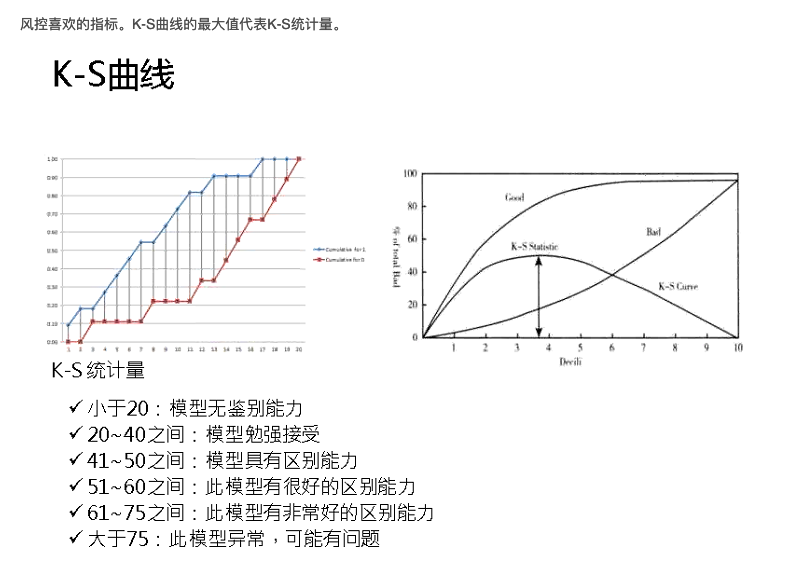# 三 GINI系数

·GINI系数:也是用于模型风险区分能力进行评估。
GINI统计值衡量坏账户数在好账户数上的的累积分布与随机分布曲线之间的面积，好账户与坏账户分布之间的差异越大，GINI指标越高，表明模型的风险区分能力越强。

GINI系数的计算步骤如下：
1. 计算每个评分区间的好坏账户数。
4. 计算出图中阴影部分面积，阴影面积占直角三角形ABC面积的百分比，即为GINI系数。

# 四 Lift , Gain

Lift图衡量的是，与不利用模型相比，模型的预测能力“变好”了多少，lift(提升指数)越大，模型的运行效果越好。
Gain图是描述整体精准度的指标。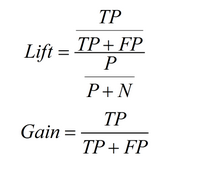1. 根据学习器的预测结果（注意，是正例的概率值，非0/1变量）对样本进行排序（从大到小）-----这就是截断点依次选取的顺序
2. 按顺序选取截断点，并计算Lift和Gain ---也可以只选取n个截断点，分别在1/n，2/n，3/n等位置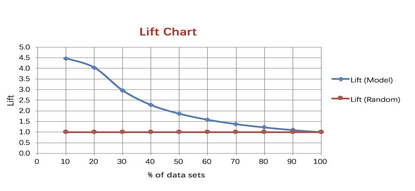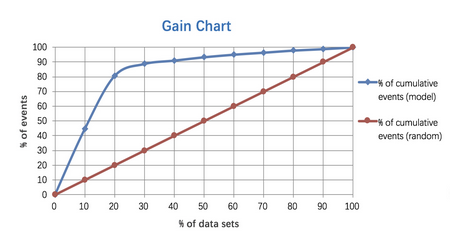# 五 模型稳定度指标PSI

PSI = sum（（实际占比-预期占比）* ln（实际占比/预期占比））

PS：除了按概率值大小等距十等分外，还可以对概率排序后按数量十等分，两种方法计算得到的psi可能有所区别但数值相差不大。

ks值 机器学习 相关内容

2018-06-27 15:56:49 sinat_36264666 阅读数 642
• ###### Kubernetes 实战系列

Kubernetes（KS8） 实战系列教程，该课程将对Kubernetes的Node、Pod、Replication Controller、Service等基本核心概念进行讲解，这些组件共同构成了Kubernetes的系统框架和计算模型。通过对它们进行灵活的组合，用户就可以快速、方便地对容器集群进行配置、创建和管理。

9522 人正在学习 去看看 CSDN讲师

==================分割线=====================

## 1.性能度量（Performance Measure）

• 性能度量是衡量模型泛化能力的数值评价标准，反映了当前问题（任务需求）；
• 使用不同的性能度量可能会导致不同的评判结果；
• 关于模型“好坏”的判断，不仅取决于算法和数据，还取决于当前任务需求；

========================分割线==================

# 2.回归（Regression）问题的评估指标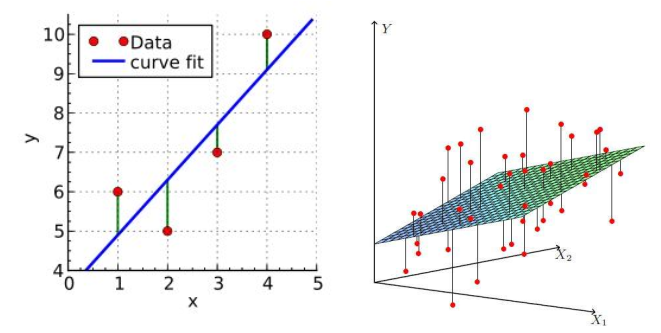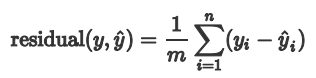==================分割线======================

## 2.1 平均绝对误差 MAE(Mean Absolute Error)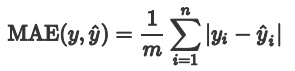MAE虽能较好衡量回归模型的好坏，但是绝对值的存在导致函数不光滑，在某些点上不能求导，可以考虑将绝对值

===================分割线======================

## 2.2 均方误差 MSE(Mean Square Error)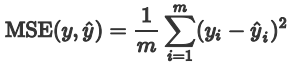===================分割线======================

## 2.3 均方根误差 RMSE （Root Mean Square Error）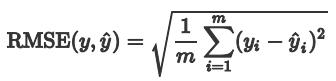===================分割线======================

## 2.4 决定系数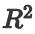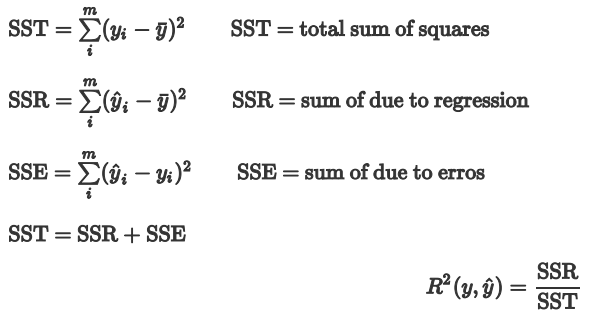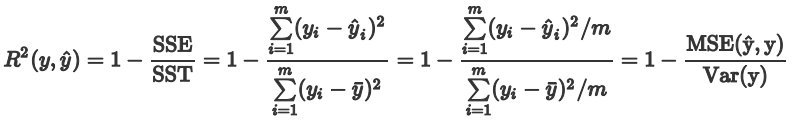===================分割线======================

## 2.5 解决评估指标鲁棒性问题

• 剔除异常值

• 使用误差的分位数来代替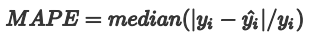MAPE是一个相对误差的中位数，当然也可以使用别的分位数。

=======================分割线========================

# 3.分类（Regression）算法评估指标

## 3.1 精度 （Acc）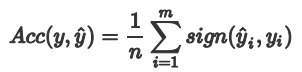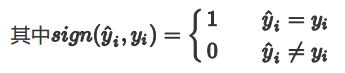• 对于有倾向性的问题，往往不能用精度指标来衡量。比如，判断空中的飞行物是导弹还是其他飞行物，很显然为了减少损失，我们更倾向于相信是导弹而采用相应的防护措施。此时判断为导弹实际上是其他飞行物与判断为其他飞行物实际上是导弹这两种情况的重要性是不一样的；
• 对于样本类别数量严重不均衡的情况，也不能用精度指标来衡量。比如银行客户样本中好客户990个，坏客户10个。如果一个模型直接把所有客户都判断为好客户，得到精度为99%，但这显然是没有意义的。
• 又比如某个地区某天地震的预测，假设我们有一堆的特征作为地震分类的属性，类别只有两个：0-不发生地震、1-发生地震。一个不加思考的分类器，对每一个测试用例都将类别划分为0，那那么它就可能达到99%的精度，但真的地震来临时，这个分类器毫无察觉，这个分类带来的损失是巨大的。为什么99%的精度的分类器却不是我们想要的，因为这里数据分布不均衡，类别1的数据太少，完全错分类别1依然可以达到很高的精度却忽视了我们关注的东西。

===================分割线======================

## 3.2 混淆矩阵（Confusion Matrix）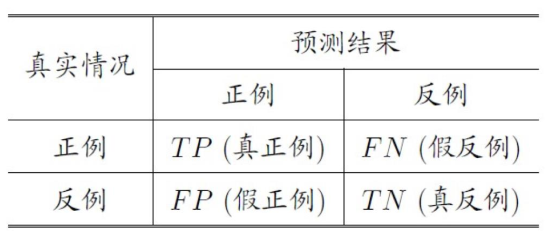• True Positive(真正, TP)：将正类预测为正类数；
• True Negative(真负 , TN)：将负类预测为负类数；
• False Positive(假正, FP)：将负类预测为正类数，误报 (Type I error)；
• False Negative(假负 , FN)：将正类预测为负类数，漏报 (Type II error)；

===================分割线======================

## 3.3 查准率（准确率） Precision

Precision 是分类器预测的正样本中预测正确的比例，取值范围为[0,1]，取值越大，模型预测能力越好。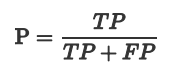===================分割线======================

## 3.4查全率（召回率）Recall

Recall 是分类器所预测正确的正样本占所有正样本的比例，取值范围为[0,1]，取值越大，模型预测能力越好。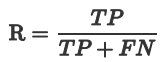• 地震的预测

• 嫌疑人定罪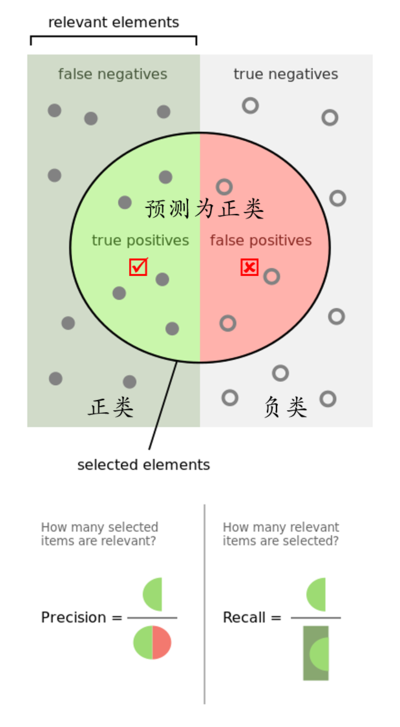===================分割线======================

## 3.5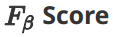PrecisionRecall 是互相影响的，理想情况下肯定是做到两者都高，但是一般情况下Precision高、Recall 就低，Recall 高、Precision就低。为了均衡两个指标，我们可以采用PrecisionRecall的加权调和平均（weighted harmonic mean）来衡量，即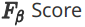公式如下：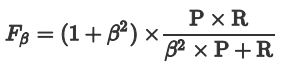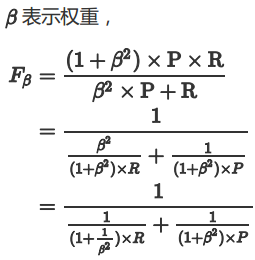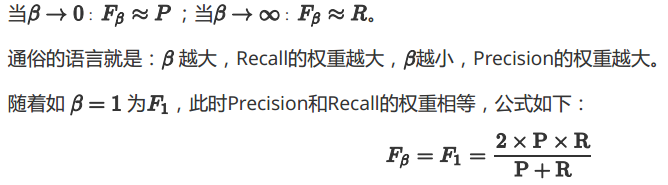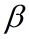>1时查全率有更大影响；<1时差准率有更大影响。由于无法直观反映数据的情况，同时业务含义相对较弱，实际工作用到的不多。

===================分割线======================

## 3.6 ROC 和 AUC

### 3.6.1 ROC

ROC曲线为 FPR TPR 之间的关系曲线，这个组合以 FPR TPR，即是以代价 (costs) 对收益 (benefits)，显然收益越高，代价越低，模型的性能就越好。

• x 轴为假阳性率（FPR）：在所有的负样本中，分类器预测错误的比例；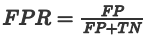• y 轴为真阳性率（TPR）：在所有的正样本中，分类器预测正确的比例（等于Recall）；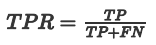TPR越高越好。而尽量降低没病误诊为有病的人数，也就是FPR越低越好。

TPR应该会很高，但是FPR也就相应地变高。最极端的情况下，他把所有的样本都看做有病，那么TPR达到1FPR也为1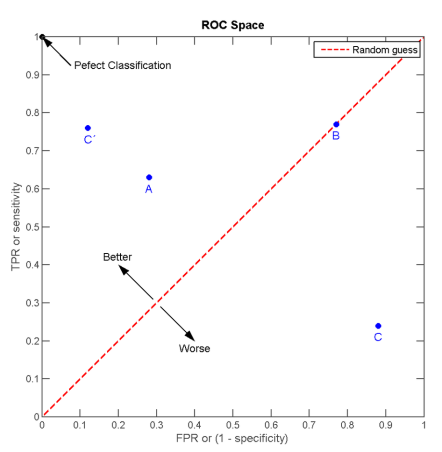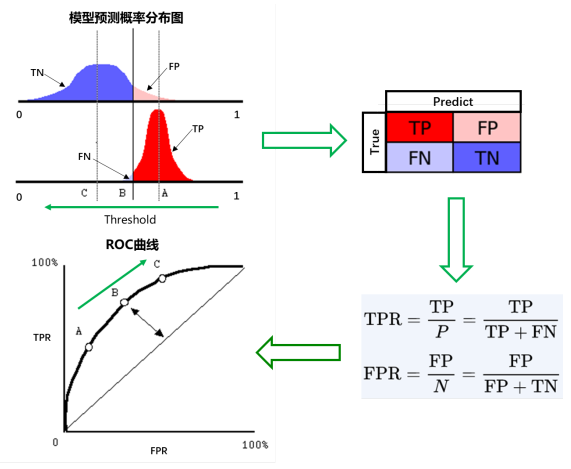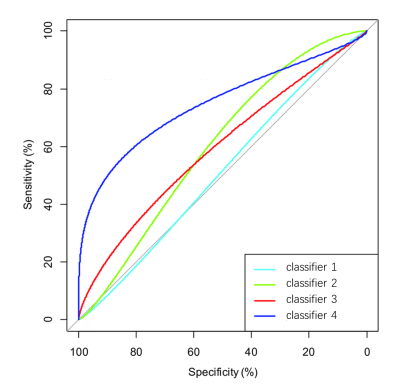===================分割线======================

### 3.6.2 AUC

AUC定义：
AUC 值为 ROC 曲线所覆盖的区域面积，显然，AUC越大，分类器分类效果越好。
• AUC = 1，是完美分类器。
• 0.5 < AUC < 1，优于随机猜测。有预测价值。
• AUC = 0.5，跟随机猜测一样（例：丢铜板），没有预测价值。
• AUC < 0.5，比随机猜测还差；但只要总是反预测而行，就优于随机猜测。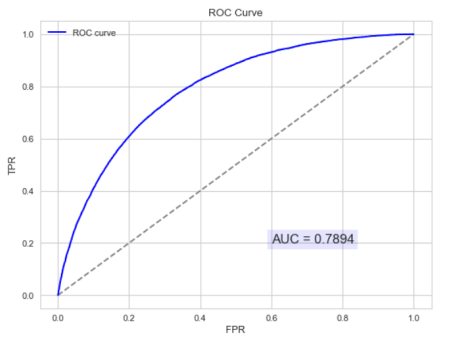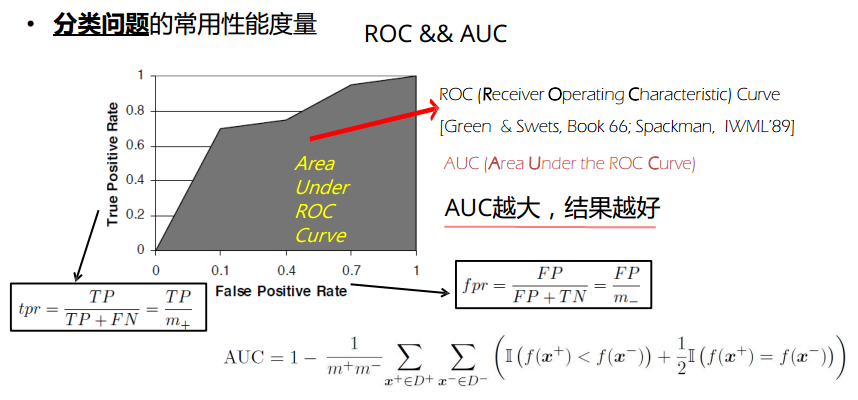AUC的物理意义
AUC的物理意义正样本的预测结果大于负样本的预测结果的概率。所以AUC反应的是分类器对样本的排序能力。另外值得注意的是，AUC对样本类别是否均衡并不敏感，这也是不均衡样本通常用AUC评价分类器性能的一个原因。

• 1. AUC更多的是关注对计算概率的排序，关注的是概率值的相对大小，与阈值和概率值的绝对大小没有关系。
• 2. AUC只关注正负样本之间的排序，并不关心正样本内部，或者负样本内部的排序。这也体现了AUC的本质：任意个正样本的概率都大于负样本的概率的能力 。

AUC的计算：

• 1AUCROC曲线下的面积，那我们直接计算面积可得。面积为一个个小的梯形面积（曲线）之和。计算的精度与阈值的精度有关。
• 2：根据AUC的物理意义，我们计算正样本预测结果大于负样本预测结果的概率。取n1*n0(n1为正样本数，n0为负样本数)个二元组，比较score（预测结果），最后得到AUC。时间复杂度为O(N*M)
• 3：我们首先把所有样本按照score排序，依次用rank表示他们，如最大score的样本，rank=n (n=n0+n1，其中n0为负样本个数，n1为正样本个数)，其次为n-1。那么对于正样本中rank最大的样本，rank_max，有n1-1个其他正样本比他score,那么就有(rank_max-1)-(n1-1)个负样本比他score小。其次为(rank_second-1)-(n1-2)。最后我们得到正样本大于负样本的概率为：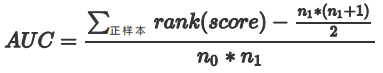计算复杂度为O(N+M)

ROC曲线只与横坐标 (FPR) 和 纵坐标 (TPR) 有关系，我们知道：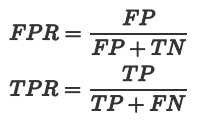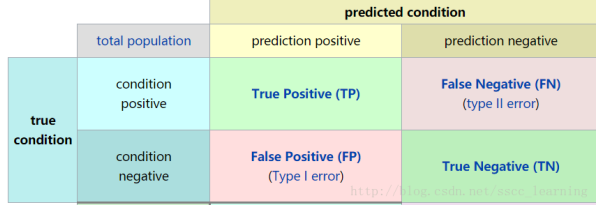===================分割线======================

## 3.7 KS Kolmogorov-Smirnov

KS值是在模型中用于区分预测正负样本分隔程度的评价指标，一般应用于金融风控领域。与ROC曲线相似，ROC是以FPR作为横坐标，TPR作为纵坐标，通过改变不同阈值，从而得到ROC曲线。而在KS曲线中，则是以阈值作为横坐标，以FPR和TPR作为纵坐标，ks曲线则为TPR-FPR，ks曲线的最大值通常为ks值。

KS值的取值范围是[0，1]。通常来说，值越大，模型区分正负样本的能力越强（一般0.3以上，说明模型的效果比较好）。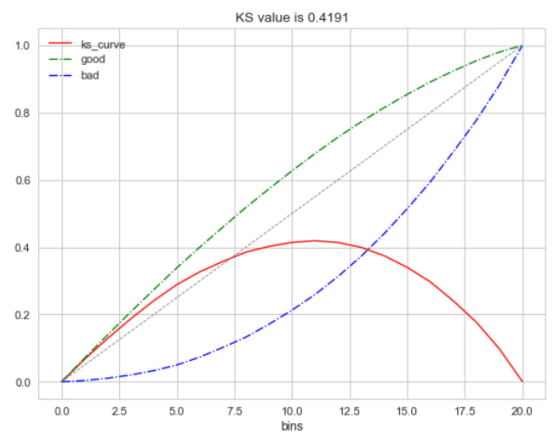===================分割线======================

# 4. 评估指标和代价函数是一家人吗？

1. 直观，可以理解
......

1. 函数光滑且可导：可用梯度下降求解极值
2. 函数为凸函数：可用梯度下降求解最优解......

===================分割线======================

# 5. 补充小知识点：micro还是macro？

## 5.1 macro方法

1. 计算出各混淆矩阵的RecallPrecision，记为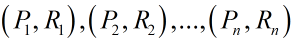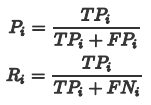2. 对各个混淆矩阵的RecallPrecision求平均，然后再根据求得的RecallPrecision计算F1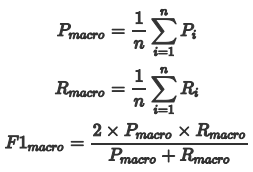===================分割线======================

## 5.2 micro方法

1. 将各混淆矩阵对应的元素进行平均，得到平均混淆矩阵：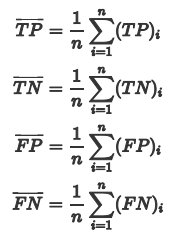2. 再基于平均混淆矩阵计算RecallPrecision，然后再根据求得的RecallPrecision计算F1

====================分割线==================

# 参考文献：

=====================END======================

ks值 机器学习 相关内容

2017-04-02 20:06:19 u011092188 阅读数 3851
• ###### Kubernetes 实战系列

Kubernetes（KS8） 实战系列教程，该课程将对Kubernetes的Node、Pod、Replication Controller、Service等基本核心概念进行讲解，这些组件共同构成了Kubernetes的系统框架和计算模型。通过对它们进行灵活的组合，用户就可以快速、方便地对容器集群进行配置、创建和管理。

9522 人正在学习 去看看 CSDN讲师

在机器学习中我们把数据分为测试数据训练数据

以下是维基百科中的解释：

• Training set: A set of examples used for learning, which is to fit the parameters [i.e., weights] of the classifier.

• Validation set: A set of examples used to tune the parameters [i.e., architecture, not weights] of a classifier, for example to choose the number of hidden units in a neural network.

• Test set: A set of examples used only to assess the performance [generalization] of a fully specified classifier.

ks值 机器学习 相关内容

2019-05-23 14:22:42 a794922102 阅读数 86
• ###### Kubernetes 实战系列

Kubernetes（KS8） 实战系列教程，该课程将对Kubernetes的Node、Pod、Replication Controller、Service等基本核心概念进行讲解，这些组件共同构成了Kubernetes的系统框架和计算模型。通过对它们进行灵活的组合，用户就可以快速、方便地对容器集群进行配置、创建和管理。

9522 人正在学习 去看看 CSDN讲师

## 8.学习理论

### 1.交叉验证

#### k-折交叉验证

1. 随机将训练集 $S$ 切分成 $k$ 个不相交的子集。其中每一个子集的规模为 $m/k$ 个训练样本。这些子集为 $S_1,\cdots,S_k$

2. 对每个模型 $M_i$，我们都按照下面的步骤进行评估(evaluate):

$j=1,\cdots,k$

• $S_1\cup\cdots\cup S_{j-1}\cup S_{j+1}\cup\cdots\cup S_k$ (也就是除了 $S_j$ 之外的其他数据)，对模型 $M_i$ 得到假设 $h_{ij}$ 。接下来针对 $S_j$ 使用假设 $h_{ij}$ 进行测试，得到经验误差 $\hat\epsilon_{S_{cv}}(h_{ij})$

$\hat\epsilon_{S_{cv}}(h_{ij})$ 取平均值，计算得到的值就当作是模型 $M_i$ 的估计泛化误差（estimated generalization error）

3. 选择具有最小估计泛化误差(lowest estimated generalization error)的模型 $M_i$ 的，然后在整个训练样本集 $S$ 上重新训练该模型。这样得到的假设 (hypothesis)就可以输出作为最终结果了。

### 2.特征选择

#### 向前搜索

1. 初始化一个集合为空集 $\mathcal F=\emptyset$

2. 循环下面的过程{

(a) 对于 $i=1,\cdots,n$ 如果 $i\notin \mathcal F$，则令 $\mathcal F_i=\mathcal F\cup \{i\}$，然后使用某种交叉验证来评估特征 $\mathcal F_i$

(b) 令 $\mathcal F$ 为(a)中最佳特征子集

}

3. 整个搜索过程中筛选出来了最佳特征子集(best feature subset)，将其输出。

#### 向后搜索

$\mathcal F = \{1, ..., n\}$ ，即规模等同于全部特征开始，然后重复，每次删减一个特征，直到 $\mathcal F$ 为空集时终止。

#### 过滤器特征选择

${\rm{MI}}(x_i, y)=\sum_{x_i\in\{0, 1\}}\sum_{y\in\{0,1\}}p(x_i,y)\log\frac{p(x_i,y)}{p(x_i)p(y)}$
(上面这个等式假设了 $x_i$$y$ 都是二值化；更广泛的情况下将会超过变量的范围 。)上式中的概率$p(x_i,y)$$p(x_i)$$p(y)$ 都可以根据它们在训练集上的经验分布(empirical distributions)而推测(estimated)得到。

${\rm{MI}}(x_i,y)={\rm KL}(p(x_i,y)\,\|\,p(x_i)p(y))$

### 3.贝叶斯统计和正则化

$\theta_{\rm ML}=\arg \max_{\theta}\prod_{i=1}^{m}p(y^{(i)}|x^{(i)};\theta)$

\begin{aligned} p(S|\theta)&=\prod_{i=1}^{m}p(y^{(i)}|x^{(i)},\theta)\\ p(S,\theta)&=p(S|\theta)p(\theta)=\prod_{i=1}^{m}p(y^{(i)}|x^{(i)},\theta)p(\theta)\\ p(S)&=\int_{\theta} {p(S,\theta)}d\theta=\int_{\theta} {\left(\prod_{i=1}^{m}p(y^{(i)}|x^{(i)},\theta)p(\theta)\right)}d\theta \end{aligned}

\begin{aligned} p(\theta|S) &=\frac{p(S|\theta)p(\theta)}{p(S)}\\ &=\frac{(\prod_{i=1}^{m}p(y^{(i)}|x^{(i)},\theta))p(\theta)}{\int_{\theta} {\left(\prod_{i=1}^{m}p(y^{(i)}|x^{(i)},\theta)p(\theta)\right)}d\theta}\qquad \end{aligned}

\begin{aligned} p(y|x,S)&=\int_\theta p(y|x,\theta)p(\theta|S)d\theta\qquad \\ \end{aligned}

4 如果 $y$ 是一个离散值(discrete-valued)，那么此处的积分(integral)就用求和(summation)来替代。

$E[y|x,S]=\int_y y p(y|x,S)dy$

$\theta_{MAP}=\arg \max_\theta \prod_{i=1}^{m} p(y^{(i)}|x^{(i)})p(\theta)$

ks值 机器学习 相关内容